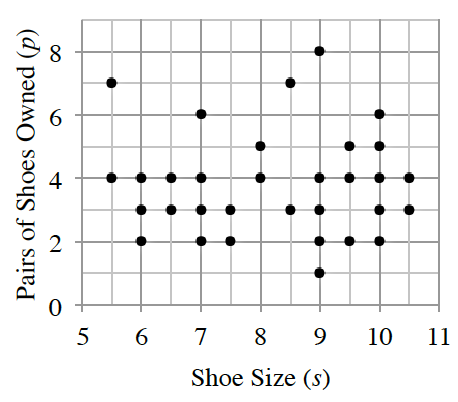### Home > GB8I > Chapter 6 Unit 7 > Lesson INT1: 6.3.3 > Problem6-109

6-109.

Make a conjecture about what $r$ is for the following scatterplot. Make a conjecture about what the $LSRL$ equation might be.

Is there any linear association between the variables?

$r ≈ 0$; Answers will vary for $LSRL$, but the average number of pairs appears to be about $3.8$ which is an $LSRL$ of $y = 3.8$.